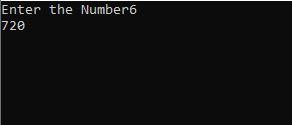## C Program to find Factorial of any Number.

C Program to find Factorial of any number.

In mathematics, the factorial of the whole number 'n' denoted by 'n!' is defined as the product of all the positive integers equal to or less than the given positive number "n"

The Formula of the factorial of any number was: -

n! = n * (n-1) * (n-2) * ....... 3 x 2 x 1.

For Example :-

5! = 5 X 4 X 3 X 2 X 1 = 120.

You can also write it as

5! = 5 X 4! = 120

Whatever we saw above was is in maths. Now we will see this through c programming. How would this formula be used in c programming?

Source Code :-

#include<stdio.h>
main()
{
int i,n,fac=1;
printf("Enter the Number");
scanf("%d",&n);
for (i=n;i>=1;--i)
fac*=i;
printf("%d",fac);
getch();
}

Explanation of the code: -
• In this code, we simply get three integer containers ( i, n, fac ) respectively. we have already defined int fac value which is 1
• Now after that, we entered a number by the user. we have placed it on the int n.
• After it, we applied a loop and the loop is running nth time until the value of the n is equal to 1.
• Every time the value of the i is decreased whenever the loop run.
• After the loop end, the product of all the numbers is stored on the int fac.
• At the end of the program, we have got the result as output.
Output: -"+y+""}else{if(A==5){c+='
•'+w+""+y+"
• "}else{if(A==6){c+='
• "+w+''+u+""+y+"
• "}else{c+='
• "+w+"